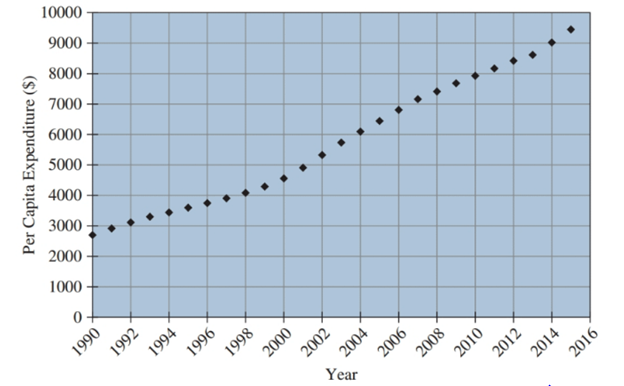# The cost of health care in the United States increases each year and is a source of current debate. The graph below page shows the per capita health care expenditure in the United States from 1990 to 2015. a. What is the total increase (in dollars) over this time period? b. What is the average yearly increase (in dollars) over this time period? c. What is the total percentage increase over this time period?### Chemistry In Focus

7th Edition
Tro + 1 other
Publisher: Cengage Learning,
ISBN: 9781337399692

#### Solutions

Chapter
Section### Chemistry In Focus

7th Edition
Tro + 1 other
Publisher: Cengage Learning,
ISBN: 9781337399692
Chapter 17, Problem 76E
Textbook Problem
1 views

## The cost of health care in the United States increases each year and is a source of current debate. The graph below page shows the per capita health care expenditure in the United States from 1990 to 2015.a. What is the total increase (in dollars) over this time period?b. What is the average yearly increase (in dollars) over this time period?c. What is the total percentage increase over this time period?Interpretation Introduction

Interpretation:

The total increase (in dollars), the average yearly increase, and the total percentage increase according to the given graph are to be determined.

Concept Introduction:

Graph is a way of representing numerical data in the form of a chart. A graph makes the analysis of data a little bit easier, for example, it is easy to detect the overall decrease or increase of a particular substance over a wide range.

A two-dimensional graph has two axis. One is the y-axis, which is the vertical axis, whereas, the other is the x-axis, which is the horizontal axis.

Certain quantities can be obtained with the help of graph analysis. These are:

Total change can be determined by subtracting the highest value from the lowest.

Average yearly changes can be found out by dividing the total change by a total number of years.

Total percentage can be determined by dividing the total change by the first value and multiplying by 100.

Yearly percentage can be calculated by dividing the total percentage change by a total number of years.

### Explanation of Solution

a) Total increase (in dollars) in health care cost over the period from 1990 to 2015.

The highest cost according to the given graph is $9500 and the lowest cost is$2800.

The total increase in cost can be found out by subtracting the highest cost by the lowest cost as follows: Total increase=$9500$2800=$6700 Hence, the total increase is$6700.

b) The average yearly increase in cost (in dollars) over the period from 1990 to 2015.

The total increase is \$6700 and the range of years according to the graph is 25 years.

Average yearly change can be found out by dividing the total increase in cost by the total number of years. It is the increase in cost per year

### Still sussing out bartleby?

Check out a sample textbook solution.

See a sample solution

#### The Solution to Your Study Problems

Bartleby provides explanations to thousands of textbook problems written by our experts, many with advanced degrees!

Get Started

Find more solutions based on key concepts
Find an article in a newspaper or magazine or on the Internet that makes claims about saturated fat, particular...

Nutrition: Concepts and Controversies - Standalone book (MindTap Course List)

How might nutritional genomics influence health care in the future?

Understanding Nutrition (MindTap Course List)

What is the difference between hydrocarbons and other organic molecules?

Biology: The Dynamic Science (MindTap Course List)

A spherically symmetric charge distribution has a charge density given by p = a/r; where a is constant. Find th...

Physics for Scientists and Engineers, Technology Update (No access codes included)

__________from a motor neuron excites a muscle fiber. a. ACh b. GABA c. calcium d. phosphate

Biology: The Unity and Diversity of Life (MindTap Course List)